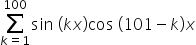Maths-
General
Easy

###Hint:

In this question, we have to find the value of. For that we will write the sum in both actual and the reverse order. Further, we will add both the equation and then solve the equation obtained to find the sum.

## Book A Free Demo+91